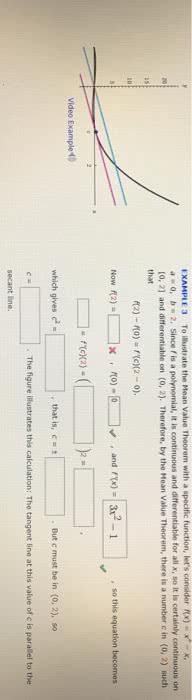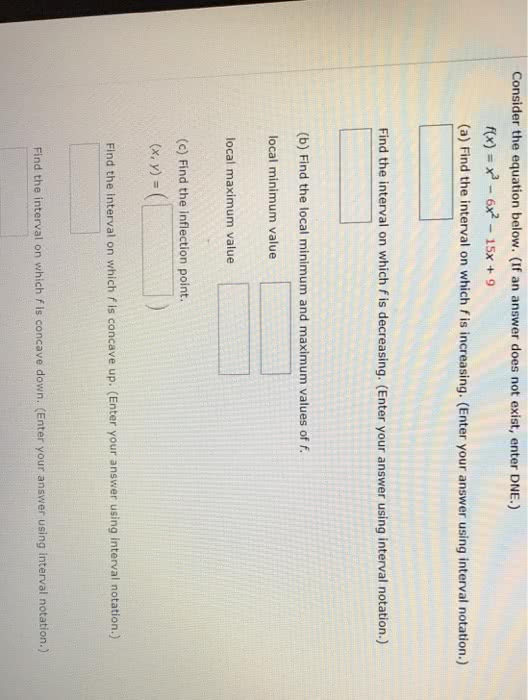1
0
watching
45
views13 Nov 2019

#EXAMPLE 3 To illustrate the Mean Value Theorem with a specific function, let's consider Nx)x ao, b 2. Since is a polynomial, it is continuous and differentiable for all x, so it is certainly continuous on [o, 21 and differentiable on (O, 2). Therefore, by the Mean Value Theorem, there is a number c in (0, 2) such that Now f(2) Video Example which gives that is, c . But c must be in (o, 2), so . The figure illustrates this calculation: The tangent line at this value of c is parallel to the